# Civil Engineering - Theory of Structures

Exercise : Theory of Structures - Section 1
36.
Two bars, one of steel and the other of copper having areas of cross-sections As and Ac, coefficient of expansion αs and αc and Young's Modulii Es and Es are rigidly connected together at the ends and subjected to temperature change of t°. If the length of the bars initially is L, the final extension δ of the two bars at t° temperature is given by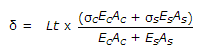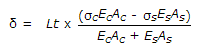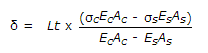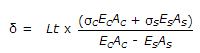Explanation:
No answer description is available. Let's discuss.

37.
The tangential component of stress on an plane inclined θ° to the direction of the force, may be obtained by multiplying the normal stress by
sin θ
cos θ
tan θ
sin θ cos θ
sin2θ
Explanation:
No answer description is available. Let's discuss.

38.
The force in AD of the truss shown in given figure, is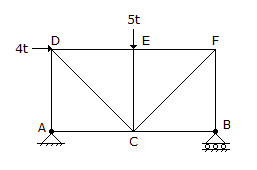4.0t compression
3.0t compression
0.5t compression
0.5t tension
zero.
Explanation:
No answer description is available. Let's discuss.

39.
There are two hinged semicircular arches A, B and C of radii 5 m, 7.5 m and 10 m respectively and each carries a concentrated load W at their crowns. The horizontal thrust at their supports will be in the ratio of
1 : 1 1/2 : 2
2 : 1 1/2 : 1
1 : 1 : 2
none of these.